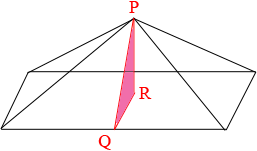SEARCH HOMEMath Central Quandaries & QueriesQuestion from Sam, a student: A right rectangular pyramid has a rectangular base measuring 18 cm by 32 cm. The height of the pyramid is 12 cm. What is the surface area?Hi Sam,

I drew a rough sketch of the pyramid and added a triangle.The surface is composed of a rectangle that has an area which is easy to calculate and four triangles. Consider the triangle that contains the points P and Q. Q is the midpoint of the base of that triangle, the length of PQ is its height and R is the midpoint of the base rectangle. What is the length QR? What is the length PQ?

PennyMath Central is supported by the University of Regina and The Pacific Institute for the Mathematical Sciences.